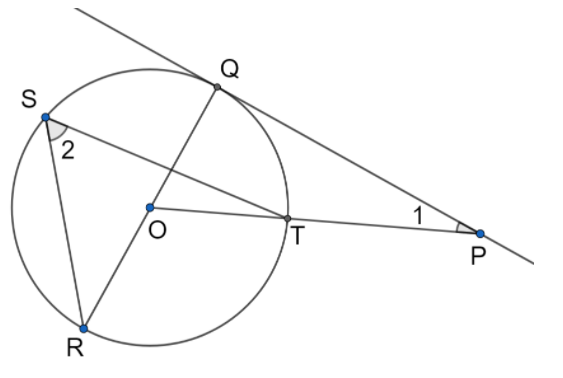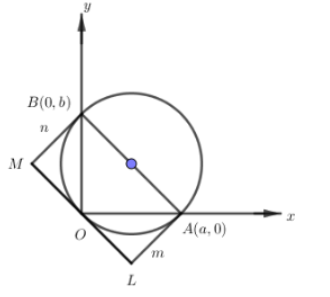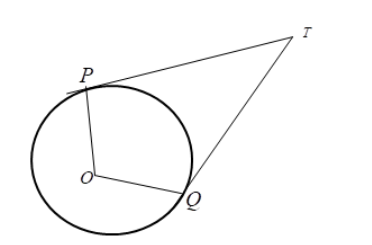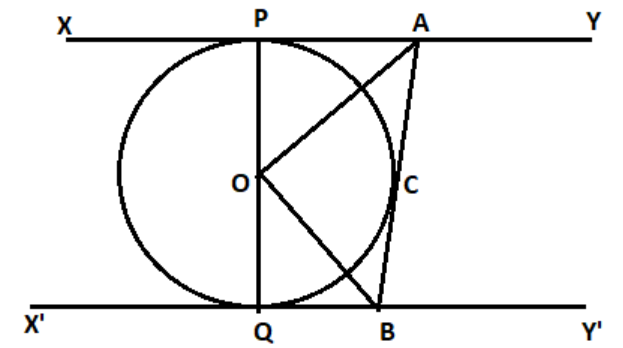Filters
Latest Questions
Mathematics
Tangent to a circle
The tangent to the circle $x^{2} + y^{2} = 9$ which is parallel to the y axis and does not lie in the third quadrant touches the circle at the point
A. $( - 3,\ 0)$
B. $(3,\ 0)$
C. $(0,\ 3)$
D. $(0,\ - 3)$
Mathematics
Tangent to a circle
Let S be the circle in the X-Y plane defined by the equation ${{x}^{2}}+{{y}^{2}}=4$. let P be a point on the circle S with both coordinates being positive. let the tangents to S at P Intersect the coordinate axes at the points M and N. then the midpoint of the segment MN must lie on the curve.
A. ${{(x+y)}^{2}}=3xy$
B. ${{x}^{\dfrac{2}{3}}}+{{y}^{\dfrac{2}{3}}}={{2}^{\dfrac{4}{3}}}$
C. ${{x}^{2}}+{{y}^{2}}=2xy$
D. ${{x}^{2}}+{{y}^{2}}={{x}^{2}}{{y}^{2}}$

Mathematics
Tangent to a circle
In the given figure, PQ is a tangent from an external point P to a circle with center O, and OP cuts the circle at T and QOR is a diameter. If $\angle POR={{130}^{\circ }}$ and S is a point on the circle, find $\angle 1+\angle 2$Mathematics
Tangent to a circle
A line meets the coordinate axes in A and B. A circle is circumscribed about the triangle OAB. If m and n are the distances of the tangent to the circle at the origin from the points A and B respectively, the diameter of the circle isA. m(m + n)
B. m + n
C. n(m + n)
D. $\dfrac{1}{2}\left( {m + n} \right)$

Mathematics
Tangent to a circle
The straight line $x+2y=1$ meets the coordinate axes at A and B. A circle is drawn through A, B and the origin. Then the sum of perpendicular distances from A and B from tangent to the circle at origin is:
A. $\dfrac{\sqrt{5}}{4}$
B. $\dfrac{\sqrt{5}}{2}$
C. $2\sqrt{5}$
D. $4\sqrt{5}$
Mathematics
Tangent to a circle
In figure if TP and TQ are the two tangents to a circle with center O so that $\angle POQ = {110^0},$ the $\angle PTQ$ is equal to.A.${60^0}$
B.${70^0}$
C.${80^0}$
D.${90^0}$
Mathematics
Tangent to a circle
Let PQ and RS be tangents at the extremities of the diameter PR of a circle of radius r. If PS and RQ intersect a point x on the circumference of the circle, then 2r equals-
A) $\sqrt {PQ.RS}$
B) $\dfrac{{PQ + RS}}{2}$
C) $\dfrac{{2PQ + RS}}{{PQ + RS}}$
D) $\sqrt {\dfrac{{P{Q^2} + R{S^2}}}{2}}$
Mathematics
Tangent to a circle
Find the diameter of the sun in Km supposing that it subtends an angle of 32’ at the eye of the observer. Given that the distance of the sun from the observer $=91\times {{10}^{6}}km$

Mathematics
Tangent to a circle
Find the length of the tangent from the point $$\left( {5,7} \right)$$ to the line $${x^2} + {y^2} - 4x - 6y + 9 = 0$$?
Mathematics
Tangent to a circle
Two circles with equal radii are intersecting at the points (0, 1) and (0, -1). The tangent at the point (0, 1) to one of the circles. Then the distance between the centres of these circles is:
A. 1
B.$\sqrt{2}$
C. 2$\sqrt{2}$
D. 2
Mathematics
Tangent to a circle
What are the tools required for constructing a tangent to a circle?
A.Ruler
B.Compass
C.Pencil
D.All the above

Mathematics
Tangent to a circle
In the figure XY and X’Y’ are two parallel tangents to a circle with centre O and another tangent AB with point of contact C intersecting XY at A and X’Y’ at B prove that $\angle {\text{AOB = 9}}{{\text{0}}^\circ}$.Prev
1
2
3
4
5